﻿ 沪港通背景下AH股溢价率影响因素研究 Research on the Influencing Factors of AH Share Premium Rate under the Background of Shanghai-Hong Kong Stock Connect

Finance
Vol.08 No.01(2018), Article ID:23411,10 pages
10.12677/FIN.2018.81003

Research on the Influencing Factors of AH Share Premium Rate under the Background of Shanghai-Hong Kong Stock Connect

Yufeng Ji, Hongli Lu

Glorious Sun School of Business and Management, Donghua University, Shanghai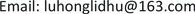Received: Dec. 18th, 2017; accepted: Jan. 4th, 2018; published: Jan. 15th, 2018ABSTRACT

This paper takes A + H cross-listed companies as research samples during the period from 2012 to 2016, and studies the spread of A + H shares of sample companies before and after the establishment of Shanghai-Hong Kong Stock Connect. The analysis of the premium rate of H shares of A shares based on liquidity differences, demand differences, investor structure differences, financing cost differences, exchange rates, market conditions and industry factors. The results show that the opening of Shanghai-Hong Kong Stock Connect has not reduced the premium of H shares of A shares but instead widened the spread of H shares of A shares. Among the factors affecting the premium rate, the effect of market liquidity is significantly positive, the impact of the demand elasticity, financing cost and exchange rate is significantly negative. And the research shows that the premium rate of the financial industry is significantly lower than the non-financial industry. Finally, in view of narrowing the price difference of A shares and accelerating the market integration, this article put forward a number of policy recommendations.

Keywords:Shanghai-Hong Kong Stock Connect System, The Premium Rate of A + H Share, Market Segmentation, Panel Data1. 引言

2014年11月17日，沪港通正式开通，意味着境内将以资本市场开放为主，进一步促进中国内地与香港资本市场的双向开放和健康发展。沪港通，即沪港股票市场交易互联互通机制，是上海证券交易所和香港联合交易所通过技术连接，允许两地投资者通过当地的证券公司或者经纪商买卖对方交易市场一定范围的股票。沪港通的开通，打开了投资者进入对方市场的通道，增加了我国境内市场的开放程度，使两地资本市场联系更加密切。

2. A + H股价差异影响因素的理论分析

2.1. 需求弹性

Li & Yan (2005)  ，Wu，Chou，Hao & Lu (2014)  ，Zhang Junming (2016)  ，曾剑宇(2014)  ，刘昕(2004)  认为需求弹性是溢价率的主要因素，境内资本市场中投资渠道较少，A股市场成为投资者的主要投资场所，需求弹性低，能接受较高的价格；而香港市场是一个较为成熟的市场，投资者除了H股，还可以投资于其他金融产品，需求价格弹性较大，所能接受的价格较低。因此，A股相对于H股出现溢价现象。

2.2. 流动性

2.3. 市场环境

A股H股价格均受到两地市场行情的影响。胡章宏，王晓坤(2008)  认为市场环境对溢价率有重要的影响。当A股牛市而H股熊市时，两地投资者将涌向A股市场，抬高A股价格的同时压低H股价格，AH股溢价率上升；情况相反时，则抬高H股价同时压低A股价格，AH股溢价率降低。

2.4. 行业因素

2.5. 投资者结构

2.6. 融资成本

2.7. 人民币汇率

3. 变量选取与模型设计

3.1. 变量的选取

3.1.1. 因变量的选择及含义

$Pr{e}_{it}=\frac{{P}_{it}^{A}-{P}_{it}^{H}}{{P}_{it}^{H}}$

$Pr{e}_{it}<0$ 时，A股相对于H股折价。

3.1.2. 自变量的选择及含义

a) 流动性指标

$Liquidit{y}_{it}=\frac{turnove{r}_{it}^{A}}{turnove{r}_{it}^{H}}$

b) 需求价格弹性指标

$Deman{d}_{it}=\frac{ts{o}_{it}^{A}}{ts{o}_{it}^{H}}$

c) 投资者结构指标

d) 融资成本指标

$C{f}_{t}=\frac{SHIBO{R}_{t}}{CNH\text{}HIBO{R}_{t}}$

e) 人民币汇率指标

f) 市场环境指标

${R}_{t}^{A}=\mathrm{ln}\left(SZ{I}_{t}\right)-\mathrm{ln}\left(SZ{I}_{t-1}\right)$

${R}_{t}^{H}=\mathrm{ln}\left(HZ{I}_{t}\right)-\mathrm{ln}\left(HZ{I}_{t-1}\right)$

g) 行业差异指标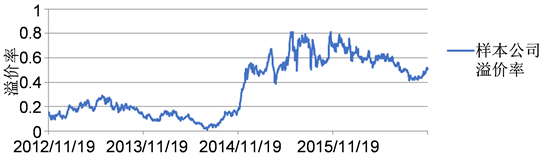数据来源：wind咨询金融终端。

Figure 1. The premium rate of Sample Firms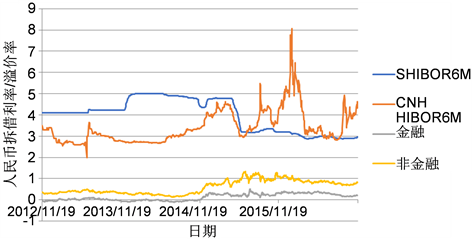数据来源：wind咨询金融终端。

Figure 2. The trend of the interest rate and premium rate of RMB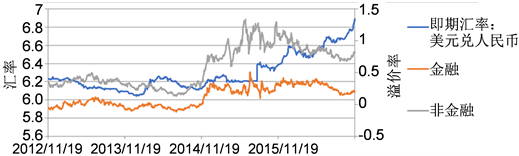数据来源：wind咨询金融终端。

Figure 3. The trend of exchange rate and premium rate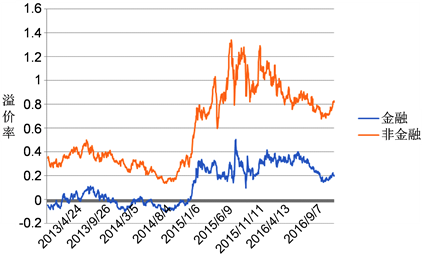数据来源：wind咨询金融终端。

Figure 4. The trend of premium rate in financial and non-financial industries

3.2. 模型的设计

$\begin{array}{l}Pr{e}_{it}=c+{\beta }_{1}Deman{d}_{it}+{\beta }_{2}Liquidit{y}_{it}+{\beta }_{3}perholdin{g}_{it}+{\beta }_{4}C{f}_{t}+{\beta }_{5}{E}_{t}\\ \text{\hspace{0.17em}}\text{\hspace{0.17em}}\text{\hspace{0.17em}}\text{\hspace{0.17em}}\text{\hspace{0.17em}}\text{\hspace{0.17em}}\text{\hspace{0.17em}}\text{\hspace{0.17em}}\text{\hspace{0.17em}}+{\beta }_{6}{R}_{t}^{A}+{\beta }_{7}{R}_{t}^{H}+{\beta }_{8}Financ{e}_{it}\end{array}$

$\begin{array}{l}Pr{e}_{it}=c+{\beta }_{1}Deman{d}_{it}+{\beta }_{2}Liquidit{y}_{it}+{\beta }_{3}perholdin{g}_{it}+{\beta }_{4}C{f}_{t}+{\beta }_{5}{E}_{t}\\ \text{\hspace{0.17em}}\text{\hspace{0.17em}}\text{\hspace{0.17em}}\text{\hspace{0.17em}}\text{\hspace{0.17em}}\text{\hspace{0.17em}}\text{\hspace{0.17em}}\text{\hspace{0.17em}}\text{\hspace{0.17em}}+{\beta }_{6}{R}_{t}^{A}+{\beta }_{7}{R}_{t}^{H}+{\beta }_{8}Financ{e}_{it}+{\beta }_{9}HG{T}_{t}\end{array}$

4. 实证检验分析

4.1. 单位根检验

4.2. 协整检验

4.3. 实证分析

4.3.1. 流动性

4.3.2. 需求弹性

4.3.3. 投资者结构

4.3.4. 融资成本

4.3.5. 人民币汇率

4.3.6. 市场特征

4.3.7. 行业因素

4.3.8. 沪港通

5. 结论与建议

5.1. 扩大投资渠道，建立套利机制

5.2. 推出金融创新，改善投机氛围

5.3. 加强金融监管，防范市场风险

5.4. 改变投资理念，倡导理性投资

A股H股价格差异部分原因在于内地与香港市场投资者结构不同，我国A股市场中个人投资者比例较大，他们缺乏专业的投资知识，不注重基本面的分析，其投资行为多偏向于投机性，并且容易出现羊群效应。个人投资者的非理性行为不利于发挥股票市场的价格发现功能，造成股价与股票价值偏离现象。而H股市场投资者主要为机构投资者，属于理性投资者，注重企业的基本面分析，股票一旦买入，偏好长期持有，投资性强。监管部门应该加强对投资者的教育，同时大力扶持民间各类合法投资公司，大力培育优质机构投资者，积极引导改变A股投资市场投资理念。

Research on the Influencing Factors of AH Share Premium Rate under the Background of Shanghai-Hong Kong Stock Connect[J]. 金融, 2018, 08(01): 26-35. http://dx.doi.org/10.12677/FIN.2018.81003

1. 1. Li, Y., Yan, D. and Greco, J. (2006) Market Segmentation and Price Differentials between A Shares and H Shares in the Chinese Stock Markets. Journal of Multinational Financial Management, 16, 232-248. https://doi.org/10.1016/j.mulfin.2005.07.003

2. 2. Wu, Q., Chou, R.K., Hao, Y. and Lu, J. (2014) Sources of Investor Sentiment and Price Deviations of Cross-Listed Shares: Evidence from China A and H Shares. School of Economic and Business Administration, Chongqing University, Chongqing.

3. 3. Zhang, J.M. (2016) The Impact of Shanghai-Hong Kong Stock Connect on the Pricing Dynamics of A-H shares.

4. 4. 曾剑宇. 金融危机以来A + B股价差变化的实证研究[J]. 时代金融, 2014(1): 245-251.

5. 5. 刘昕. 信息不对称与H股折价关系的定量研究[J]. 财经研究, 2004 (4): 39-49.

6. 6. Domowitz, L., Glen, J. and Madhavan, A. (1997) Market Segmentation and Stock Prices: Evidence from an Emerging Market. Journal of Finance, 52, 1059-1085. https://doi.org/10.1111/j.1540-6261.1997.tb02725.x

7. 7. 韩德宗. A股和H股市场软分割因素研究——兼论推出QDII的步骤和时机[J]. 商业经济与管理, 2006(3): 42-46.

8. 8. 汪珊. 我国股票市场AB股价格差异的实证研究[D]: [硕士学位论文]. 武汉: 华中科技大学, 2011.

9. 9. 王维安, 白娜. A股与H股价格差异的实证研究[J]. 华南金融研究, 2004(4): 31-39.

10. 10. 屠年松, 吴常娟.“沪港通”对中国A、H股市场分割的影响[J]. 财政金融, 2016(2): 14-20.

11. 11. 胡章宏, 王晓坤. 中国上市公司A股和H股价差的实证研究[J]. 经济研究, 2008(4): 119-131.

12. 12. 刘小宝. 基于面板数据的我国A股H股价格差异因素分析[D]: [硕士学位论文]. 南昌: 江西财经大学, 2012.